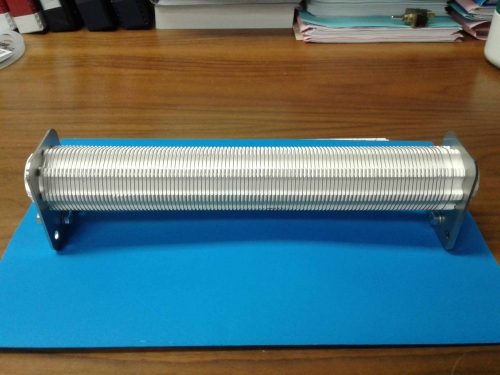Search IntMath
Close

# How do I calculate the length of wire on a tubular ceramic form? [Solved!]

### My question

I need to calculate the total length of wire required for a wire wound resistor as shown in the attached figure, given the following:

- Diameter of a single turn: D
- Number of grooves (ie number of turns on ceramic tube): n
- Pitch (distance between grooves on the x-axis): p

Does anyone have a more accurate equation for determining the total wire length?

### Relevant page

Interactive Mathematics - Learn math while you play with it!

### What I've done so farOne could approximate the total wire length by taking the circumference of a single turn and multiplying it by n, the number of turns.
This value will be less than the actual value because the pitch adds additional length.

Maybe a more accurate value will be given by: wire length = n(circumference of single turn) + (n-1)p.

X

I need to calculate the total length of wire required for a wire wound resistor as shown in the attached figure, given the following:

- Diameter of a single turn: D
- Number of grooves (ie number of turns on ceramic tube): n
- Pitch (distance between grooves on the x-axis): p

Does anyone have a more accurate equation for determining the total wire length?

I will appreciate your comments.
Relevant page

<a href="https://www.intmath.com">Interactive Mathematics - Learn math while you play with it!</a>

What I've done so farOne could approximate the total wire length by taking the circumference of a single turn and multiplying it by n, the number of turns.
This value will be less than the actual value because the pitch adds additional length.

Maybe a more accurate value will be given by: wire length = n(circumference of single turn) + (n-1)p.

## Re: How do I calculate the length of wire on a tubular ceramic form?

@Stephan: Welcome to the IntMath Forum!

Let's just consider one turn of the wire first.

Imagine this one turn of the the wire is laid out so that it's straight. The length of this wire will be the longer side of a right triangle, length piD (since the diameter is D), and height p.

What is that length?

Since we have n turns, what will be the total length of the wire?

X

@Stephan: Welcome to the IntMath Forum!

Let's just consider one turn of the wire first.

Imagine this one turn of the the wire is laid out so that it's straight. The length of this wire will be the longer side of a right triangle, length piD (since the diameter is D), and height p.

What is that length?

Since we have n turns, what will be the total length of the wire?

## Re: How do I calculate the length of wire on a tubular ceramic form?

Dear Murray,

On what you have said, the length of a single turn will then be in accordance with Pythagoras:

l = sqrt((piD)^2 + p^2)

The total length (L) of the wire will then be:

L = nl

X

Dear Murray,

On what you have said, the length of a single turn will then be in accordance with Pythagoras:

l = sqrt((piD)^2 + p^2)

The total length (L) of the wire will then be:

L = nl

## Re: How do I calculate the length of wire on a tubular ceramic form?

@Stephan: Exactly!

Good on you for trying out the math entry system.

BTW, you can enter "pi" by simply typing "pi".

X

@Stephan: Exactly!

Good on you for trying out the math entry system.

BTW, you can enter "pi" by simply typing "pi".

## Re: How do I calculate the length of wire on a tubular ceramic form?

X

Glad to hear it @jk123

## Re: How do I calculate the length of wire on a tubular ceramic form?

Thanks for post!

X

Thanks for post!

You need to be logged in to reply.

## Related Basic Algebra questions

• Addition and Subtraction of Algebraic Expressions [Pending...]
simplify −3[−5(m − 2n) + 4n] + sqrt(4n)
• Re: simple interest and compound interest [Pending...]
Tell me what your lesson notes or textbook say about Simple and Compound Interest!
• Manipulating algebra fractions [Solved!]
when solving the right hand side of an equation (fluid flow ) from sqrt(25/(1-(x^4/0.1^4))) goes to -sqrt(25/(1-10000X^4)) this...
• Absolute value [Solved!]
If x = -|w|, which of the following MUST be true A. x=-w B. x=w C. x^2=w D. x^2=w^2 E....
• order of operations [Solved!]
As the kids always say - this is probably a stupid question - but how...